# NPV

The correct NPV formula in Excel uses the NPV function to calculate the present value of a series of future cash flows and subtracts the initial investment.

### Net Present Value

For example, project X requires an initial investment of \$100 (cell B5).

1. We expect a profit of \$0 at the end of the first period, a profit of \$50 at the end of the second period and a profit of \$150 at the end of the third period.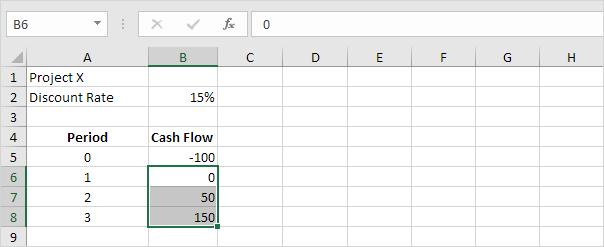2. The discount rate equals 15%.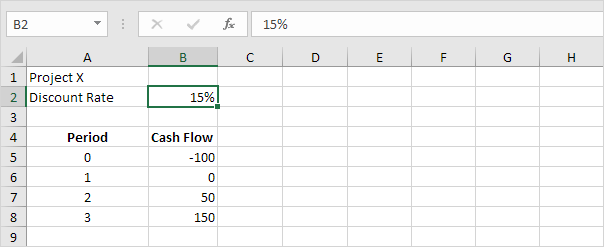Explanation: this is the rate of return of the best alternative investment. For example, you could also put your money in a high-yield savings account at an interest rate of 15%.

3. The NPV formula below calculates the net present value of project X.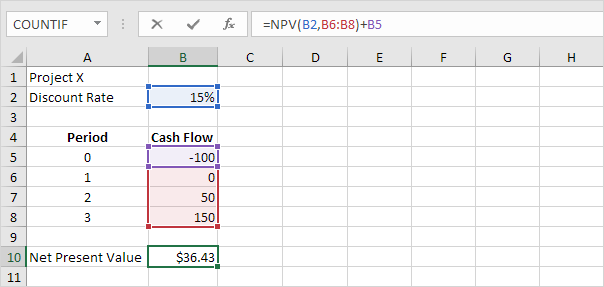Explanation: a positive net present value indicates that the project’s rate of return exceeds the discount rate. In other words, it's better to invest your money in project X than to put your money in a high-yield savings account at an interest rate of 15%.

4. The NPV formula below calculates the net present value of project Y.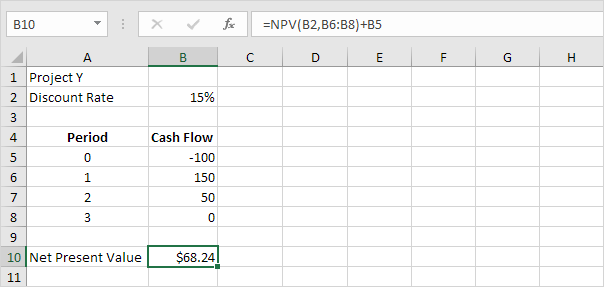Explanation: the net present value of project Y is higher than the net present value of project X. Therefore, project Y is a better investment.

### Understanding the NPV function

The NPV function simply calculates the present value of a series of future cash flows. This is not rocket science.

1. For example, project A requires an initial investment of \$100 (cell B5). We expect a profit of \$0 at the end of the first period, a profit of \$0 at the end of the second period and a profit of \$152.09 at the end of the third period.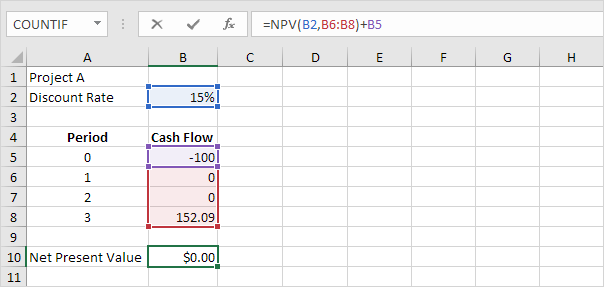Explanation: a net present value of 0 indicates that the project generates a rate of return equal to the discount rate. In other words, both options, investing your money in project A or putting your money in a high-yield savings account at an interest rate of 15%, yield an equal return.

2. We can check this. Assume you put \$100 into a bank. How much will your investment be worth after 3 years at an annual interest rate of 15%? The answer is \$152.09.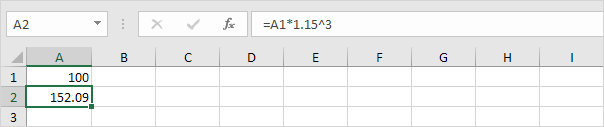3. The NPV function simply calculates the present value of a series of future cash flows.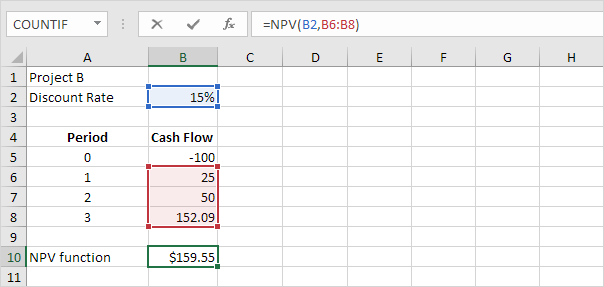4. We can check this. First, we calculate the present value (pv) of each cash flow. Next, we sum these values.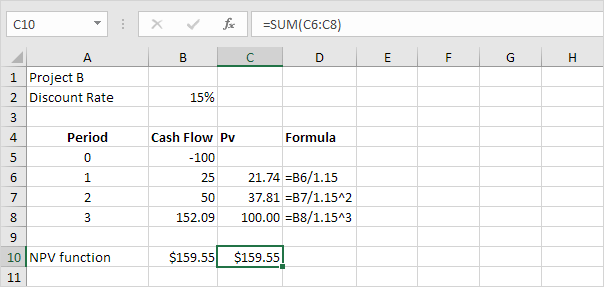Explanation: \$152.09 in 3 years is worth \$100 right now. \$50 in 2 years is worth 37.81 right now. \$25 in 1 year is worth \$21.74 right now. Would you trade \$159.55 for \$100 right now? Of course, so project B is a good investment.

5. The NPV formula below calculates the net present value of project B.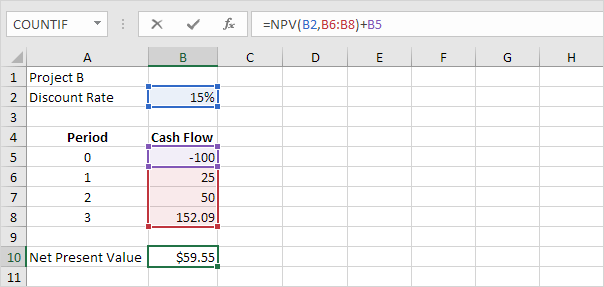Explanation: project B is a good investment because the net present value (\$159.55 - \$100) is greater than 0.

Go to Next Chapter: Statistical Functions